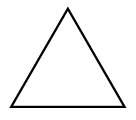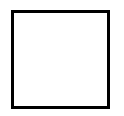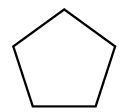### Home > CC1 > Chapter 1 > Lesson 1.1.2 > Problem1-12

1-12.

Consider the first three figures of the pattern below.Figure $1$Figure $2$Figure $3$

1. On your own paper, draw what Figure $4$ of this pattern should look like.

How many sides are in each figure shown?
How many would you expect in the next figure?

2. Using words, describe what Figures $5$ and $6$ should look like.

If Figure $4$ has $6$ sides of the same length, how many sides should the next figures have?

Figure $5$ should have seven sides of the same length.
Figure $6$ should have eight sides of the same length.

3. Using words, describe how the pattern is changing.

• How is one figure different from the previous one?
Think about how you answered part (b) and try to generalize this.